For a full list of BASHing data blog posts see the index page.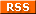# Another surprising AWK trick

This trick was nicely demonstrated in a solution to a Stack Overflow question back in 2015. Given the string:

no change of listener transaction id for last 0 checks (rid=971489 lid=970863)

how to get the difference between the "rid" and "lid" numbers? One of the posted answers was remarkably simple: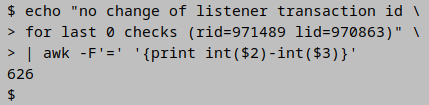And I can make that even simpler: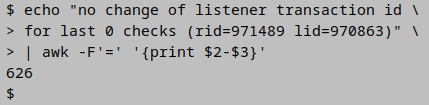At first glance this makes no sense, because field 2 in the string is "971489 lid" and field 3 is "970863)":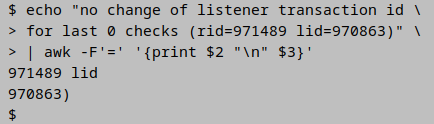So why is AWK ignoring everything but the numbers in returning "626"? Because "Strings are converted to numbers and numbers are converted to strings, if the context of the awk program demands it". In this case AWK is told to subtract field 3 from field 2. Subtraction being a numbers operation, AWK treats the strings in the fields as numbers, and since " lid" and ")" aren't numbers, they're ignored.

This string-as-number trick only works, however, if the string begins with a number. Embedded numbers are treated as non-numbers: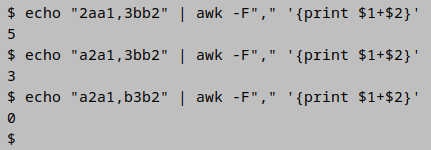When operating on numbers that don't lead the strings, it's best to use the FPAT method explained in a previous BASHing data post: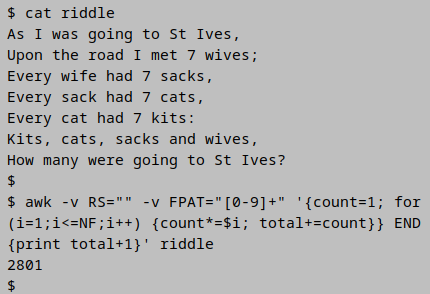awk -v RS="" -v FPAT="[0-9]+" '{count=1; for (i=1;i<=NF;i++) {count*=\$i; total+=count}} END {print total+1}' riddle

Many people answer this famous riddle with "one", because only one entity (the narrator) is known to be going to St Ives. Here I treat the riddle as an arithmetic problem whose answer is the sum of 7 wives, 49 sacks, 343 cats, 2401 kits and 1 narrator, or 2801.

Setting the record separator RS to an empty string (-v RS="") tells AWK to treat the whole "riddle" file as a single line. The fields in the line are defined by their content, namely 1 or more digits (-v FPAT="[0-9]+").

As AWK works through each field in the line (for (i=1;i<=NF;i++)) it does 2 operations. The first is to increment the variable "count" by multiplying it by the field contents (count*=\$i); "count" has previously been set to 1 as a starting value (count=1). The second operation is to increment the variable "total" by adding to it the current value of "count" (total+=count). In the END statement after the line has been processed, AWK prints the final value of "total" plus 1, for the narrator.

Last update: 2019-12-06
The blog posts on this website are licensed under a